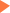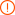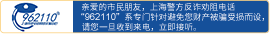# 盘点整理GMAT数学考试常用公式

2014年04月15日14:01 来源：小站整理## GMAT备考资料免费领取 请选择资料！

(a+b)(a-b)=a²-b² (a+b)²=a²+2ab+b² (a-b)²=a²-2ab+b²

(a+b)³=a³+3a²b+3ab²+b³ (a-b)³=a³-3a²b+3ab²-b³

Simple Interest:利息Interest=本金Principal*时间Time*利率Rate。

Compound Interest:A=(1+R)n;A为本利和，P为本金,R为利率,n为期数。

Discount=CostRate of Discount *Distance=Speed*Time

Pythagorean Theorem(勾股定理):直角三角形(right triangle)两直角边(legs)的平方和等于斜边 (hypotenuse)的平方。

Perimeter Area

Triangle C=三边之和 S=(底×高)/2

Square C=边长×4 S=边长的平方

Rectangle C=(长+宽)×2 S=长×宽

Parallelogram C=(长+宽)×2 S=底×高

Trapezoid C=四边之和 S=(上底+下底)×高/2

Rhombus C=边长×4 S=两条对角线之积的1/2

Circle C=2πr=πd S=πr2

### 专题推荐未输入验证码未输入验证码未输入验证码

## GMAT30天热搜沪公网安备 31010602002658号